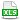HomeTechniques and Tips@RISK DistributionsCombining Frequency and Severity

# 3.13. Combining Frequency and Severity

Applies to: @RISK 5.x–7.x

I have a risk that may or may not occur, or it might occur a variable number of times. But the impact or severity of each occurrence is a probability distribution, not a fixed number. How can I model this in @RISK?

The RiskCompound function, available in @RISK 5.0 and later, is the solution. It takes two arguments: a discrete function for frequency or probability, and a discrete or continuous function to govern severity or impact. (Two additional arguments are optional; see How are the deductible and limit applied, below.)

Suppose the impact or severity is according to RiskNormal(100,10). If you want to say that the risk may or may not occur, and has 40% probability of occurrence, code it this way:

=RiskCompound(RiskBinomial(1,0.4), RiskNormal(100,10))

(For more about a risk that can occur only zero times or one time, see Event or Operational Risks.)

If you want to say that the risk could occur a variable number of times, choose one of the discrete distributions for frequency. For example, if you choose a Poisson distribution with mean 1.4 for the distribution of possible frequencies, then your complete RiskCompound function would be

=RiskCompound(RiskPoisson(1.4), RiskNormal(100,10))

In any iteration where the frequency is greater than 1, @RISK will draw multiple random numbers from the severity distribution and add them up to get the value of the RiskCompound for that iteration. (There is no way to get at the individual severity values that were drawn within one iteration.)

Must frequency and severity be @RISK distributions, or can they be references to cells that contain formulas?

You can embed the frequency and severity distributions within RiskCompound( ), as shown above, or to use cell references for frequency and severity and have those distributions in other cells. There are two caveats:

• Performance: If your frequency is large, or if you have many RiskCompound functions, your simulation will run faster — possibly much faster — if you embed the actual severity distribution within the RiskCompound( ). Using a cell reference for the frequency distribution doesn't hurt performance. (The attached CompoundExploration.xls uses cell references to make the discussion easier to follow, but it is a very small model and so performance is not a concern.)

• Calculation: If the severity argument is a cell reference, and the referenced cell contains an @RISK distribution, then the severity will be evaluated multiple times in an iteration, just as if the severity were physically embedded in the RiskCompound( ) function. For instance, suppose that the severity argument points to a cell that contains a RiskTriang( ) distribution, either alone or within a larger formula. If the frequency distribution has a value of 12 in a given iteration, then the referenced formula will be re-evaluated 12 times during that iteration, and the 12 values added together will be the value of the RiskCompound( ).

But if the referenced cell does not contain any @RISK distributions, it will be evaluated only once every iteration, even if the cell contains a formula that ultimately refers to an @RISK distribution. For example, consider the function =RiskCompound(F11,S22), and suppose that on one particular iteration the frequency value in F11 is 12. If the severity cell S22 contains a formula such as =RiskNormal(B14,B15)+B16*B17, it will be evaluated 12 times during this iteration, and the value of the RiskCompound will be the sum of those twelve values. But if the severity cell S22 contains a formula such as =LOG(B19), and B19 contains a RiskNormal( ) function, the formula will be evaluated only once in his iteration, and the value of the RiskCompound( ) for this iteration will be 12 times the value of that formula. You can think of it this way: RiskCompound( ) will drill through one level of cell referencing to find distributions, but only one level.

What if the frequency distribution is a continuous distribution? How does @RISK decide how many severity values to add up?

"Frequency" implies a number of occurrences, which implies a whole number (0 or a positive integer). Therefore we recommend a discrete distribution, returning whole numbers, for the frequency. But if you use a continuous distribution, or a discrete distribution returning non-integers, @RISK will truncate the value to an integer.

For example, if your frequency distribution returns a value of 3.7, @RISK will draw three values from the severity distribution, not four.

How are the deductible and limit applied in a RiskCompound( ) function? Is it on a per-occurrence or an aggregate basis?

RiskCompound( ) takes up to four arguments:

RiskCompound(dist1, dist2, deductible, limit)

Both deductible and limit are applied per occurrence. For example, suppose that the frequency distribution dist1 has a value of 6 in a particular iteration. Then the severity distribution dist2 will be drawn six times, and deductible and limit will be applied to each of the six.

The limit argument to RiskCompound( ) is meant to be the actual maximum payout or exposure per occurrence. If the actual maximum payout is the policy limit minus the deductible, then you should use the actual maximum payout for the fourth argument to the RiskCompound( ) function.

For each sample drawn from dist2, out of the multiple samples during an iteration, the result returned is
MIN( limit, MAX( sample - deductible, 0 ) )

In words:
1. If sample is less than or equal to deductible, zero is returned.
2. If sample is greater than deductible and (sample minus deductible) is less than limit, (sample minus deductible) is returned.
3. If (sample minus deductible) is greater than or equal to limit, limit is returned.

Again, limit and deductible are applied to each of the samples of dist2 that are drawn during a given iteration. Then the values of all the occurrences are summed, and the total is recorded as the value of the RiskCompound( ) function for that iteration. (It's not possible to get details of the individual occurrences within an iteration.)

You can download the attached workbook to try various possibilities for RiskCompound.

•CompoundExploration.xls